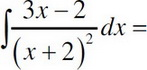# AP Calculus AB Practice Test 23

### Test Information10 questions20 minutes

Calculator Disallowed

1. Find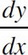if 2y2 - 6y = x4 + 2x3 - 2x - 5 at (1, 1).

2.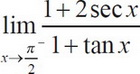3.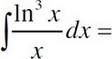4. Find the volume of the region formed by the curve y = x2 the x-axis, and the line x = 3 when revolved around the y-axis.

5.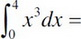6. Is the function f(x) =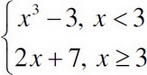continuous at x = 3? If not, what is the discontinuity?

7. Where does the curve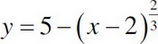have a cusp?

8.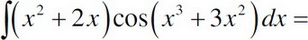9. What is the distance traveled from t = 0 to t = 4 given the position function, x(t) = 2t3 - 9t2 + 12t + 13?

10.